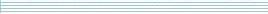| home      | people     | research     | publications    | seminars    | events     | contactCommunication Networks   | Systems Biology   | Hybrid Systems    | Machine Learning   | Dynamics & Interaction### Schools Mathematics Grand Challenge

This week's theme is theme is secret codes.

PROBLEM 10:

In this problem, you need to decipher a simple Caesar cipher, which is a form of code, and then answer the question that is encoded. You can find out more about Caesar ciphers on Wikipedia.

For this code we have shifted the letters and the digits, as if they were a long list:

abcdefghijklmnopqrstuvwxyz0123456789

For example if we shifted by 3 places, 'a' would be encoded as 'd', 'b' as 'e' and so on. At the end of the list, '6' would be encoded as '9', '7' as 'a', '8' as 'b' and '9' as 'c'.

Now decode the question below and then answer the decoded question.

E FIIOIITIV OIITW X0MGI EW QER2 FIIW EW LI HSIW WLIIT. LI OIITW A69 WLIIT. LS0 QER2 FIIW HSIW XLI FIIOIITIV OIIT

NOTE: Your answer should be the answer to the encoded question.
HINT: The most common letter in the question is 'E'.

PROBLEM 11:

Figuring out remainders can be important for all sorts of codes. When you registered you told us your age and your year in school. Let A be your age and Y be your year in school. Find a whole number so that:

• When you divide this number by 131 the remainder is Y,
• When you divide this number by A the remainder is 7.

Remember, if you've had a birthday since you registered or changed year, then you should use your age and year at the time you registered!

PROBLEM 12:

To solve this problem you must decode another message and answer the question within it. Instead of a using a Caesar Cipher, we have used a Substitution Cipher, which is a more difficult code to break. In a substitution cipher, each letter is replaced with another letter, but it is not a simple shift. For example, if we replaced "h" with "p", "e" with "f", "l" with "a" and "o" with "r" then "hello" would become "pfaar".

Substitution ciphers can be broken by looking at how common different letters are. In English the most common letter is "E". Following E, the next most common letter is "T", then "A", then "O", then "I", then "N" and then "S".

In the question, before it was encoded, the most common letters were "ETAOINS", in that order.

To solve this problem, enter your user code, then break the code and answer the question.

User code: